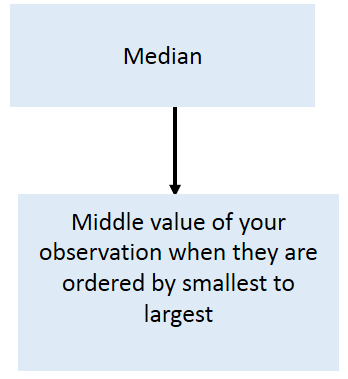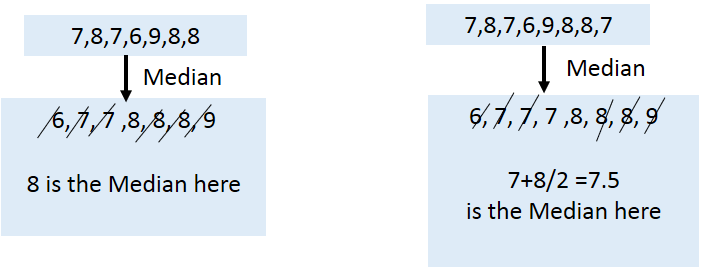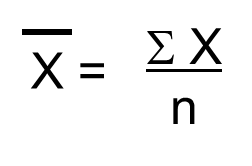### Mode, Median and Mean

In “Graphs and shapes of Distributions”  section it is explained how to summarizing a distribution of your data in terms of graphs. Now it’s time to measure the center of your distribution. Once we talk about measuring central tendency of a variable then 3 M’s come into picture.

1. Mode
2. Median
3. Mean#### Mode:

If your variable of interest is measured in nominal or ordinal (Categorical) level then Mode is the most often used technique to measure the central tendency of your data.

Finding the mode is easy. Basically, it is the value that occurs most frequently.  In other words, mode is the most common outcome. Mode is the name of the category that occurs more often.

There is a chance of having more than one mode in your variable.Here you have two modes.

##### Example:

If you still don’t understand and want to know you to calculate mode step by step then follow the below link

http://www.purplemath.com/modules/meanmode.htm

#### Median:

The second measure of central tendency is the median. The median is nothing more than the middle value of your observations when they are order from the smallest to the largest.It involves two steps:

1. Oder your cases from smallest to largest
2. Find the middle Value
• If you have odd number of cases then finding middle value is easy. Let’s think you have 5 cases. So, after ordering always 3rd position is the middle value.
• If you have even number no cases (let’s think 6 cases). In this case there is no single middle value. Then how do we calculate median? Well, we just take the average of the two middle values.
##### Example:#### Mean:

The third measure of central tendency is the most often used one, and also the one you most probably already know quite well: the mean. The mean is the sum of all the values divided by the number of observations. It is nothing but the average value.##### Now the question is When to use what measurement of central tendency?
• If data is Categorical (Nominal or Ordinal) it is impossible to calculate mean or median. So, go for mode.
• If your data is quantitative then go for mean or median. Basically, if your data is having some influential outliers or data is highly skewed then median is the best measurement for finding central tendency. Otherwise go for Mean.